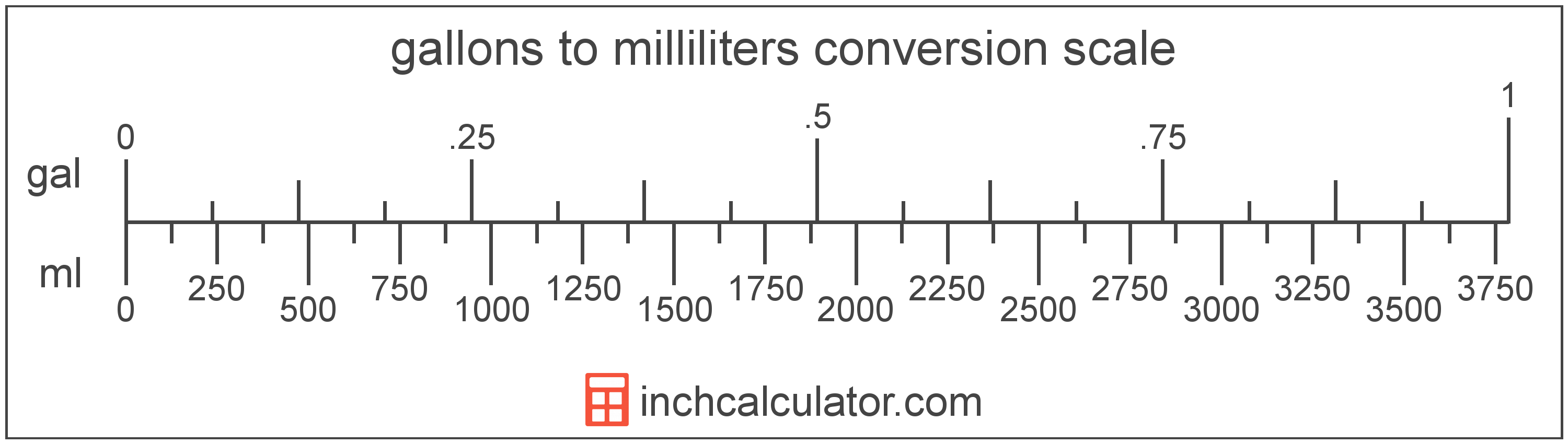# Gallons to Milliliters Converter

Enter the volume in gallons below to get the value converted to milliliters.

Results in Milliliters:1 gal = 3,785.411784 mL
1 gal = 3.785412 l

Do you want to convert milliliters to gallons?

## How to Convert Gallons to Milliliters

To convert a measurement in gallons to a measurement in milliliters, multiply the volume by the following conversion ratio: 3,785.411784 milliliters/gallon.

Since one gallon is equal to 3,785.411784 milliliters, you can use this simple formula to convert:

milliliters = gallons × 3,785.411784

The volume in milliliters is equal to the volume in gallons multiplied by 3,785.411784.

For example, here's how to convert 5 gallons to milliliters using the formula above.
milliliters = (5 gal × 3,785.411784) = 18,927.05892 mL### How Many Milliliters Are in a Gallon?

There are 3,785.411784 milliliters in a gallon, which is why we use this value in the formula above.

1 gal = 3,785.411784 mL

## What is a Gallon?

The US liquid gallon is a unit of fluid volume equal to four quarts, eight pints, or sixteen cups. The US liquid gallon should not be confused with the US dry gallon or the imperial gallon, which are different units of measure.

The gallon is a US customary unit of volume. Gallons can be abbreviated as gal; for example, 1 gallon can be written as 1 gal.

## What is a Milliliter?

The milliliter is a unit of volume equal to 1 cubic centimeter, 1/1,000 of a liter, or about 0.061 cubic inches.

The milliliter is an SI unit of volume in the metric system. In the metric system, "milli" is the prefix for thousandths, or 10-3. A milliliter is sometimes also referred to as a millilitre. Milliliters can be abbreviated as mL, and are also sometimes abbreviated as ml or mℓ. For example, 1 milliliter can be written as 1 mL, 1 ml, or 1 mℓ.

Since one milliliter is equivalent to one cubic centimeter, milliliters are sometimes expressed using the abbreviation for a cubic centimeter (cc) for things such as medical dosages or engine displacements.

## Gallon to Milliliter Conversion Table

Table showing various gallon measurements converted to milliliters.
Gallons Milliliters
0.001 gal 3.7854 mL
0.002 gal 7.5708 mL
0.003 gal 11.36 mL
0.004 gal 15.14 mL
0.005 gal 18.93 mL
0.006 gal 22.71 mL
0.007 gal 26.5 mL
0.008 gal 30.28 mL
0.009 gal 34.07 mL
0.01 gal 37.85 mL
0.02 gal 75.71 mL
0.03 gal 113.56 mL
0.04 gal 151.42 mL
0.05 gal 189.27 mL
0.06 gal 227.12 mL
0.07 gal 264.98 mL
0.08 gal 302.83 mL
0.09 gal 340.69 mL
0.1 gal 378.54 mL
0.2 gal 757.08 mL
0.3 gal 1,136 mL
0.4 gal 1,514 mL
0.5 gal 1,893 mL
0.6 gal 2,271 mL
0.7 gal 2,650 mL
0.8 gal 3,028 mL
0.9 gal 3,407 mL
1 gal 3,785 mL

## References

1. National Institute of Standards and Technology, Specifications, Tolerances, and Other Technical Requirements for Weighing and Measuring Devices, Handbook 44 - 2019 Edition, https://nvlpubs.nist.gov/nistpubs/hb/2019/NIST.HB.44-2019.pdf
2. National Institute of Standards and Technology, Specifications, Tolerances, and Other Technical Requirements for Weighing and Measuring Devices, Handbook 44 - 2019 Edition, https://nvlpubs.nist.gov/nistpubs/hb/2019/NIST.HB.44-2019.pdf